# Expressions and Equations INTRODUCTION TO EXPRESSIONS AND EQUATIONS

• Slides: 7Expressions and Equations INTRODUCTION TO EXPRESSIONS AND EQUATIONS 6 TH GRADE CHRISTIAN BRYAN OCTOBER 17 TH, 2012What is an Algebraic Expression? � An algebraic expression is an expression made up of constants, variables, and a number of algebraic operations, like addition, subtraction, multiplication and division. � A constant is a number on its own, or sometimes a letter such as a, b or c to stand for a fixed number. � A variable is a symbol for a number we don't know yet. It is usually a letter like x or y. � Operations or operators are “-, +, x, /” signs.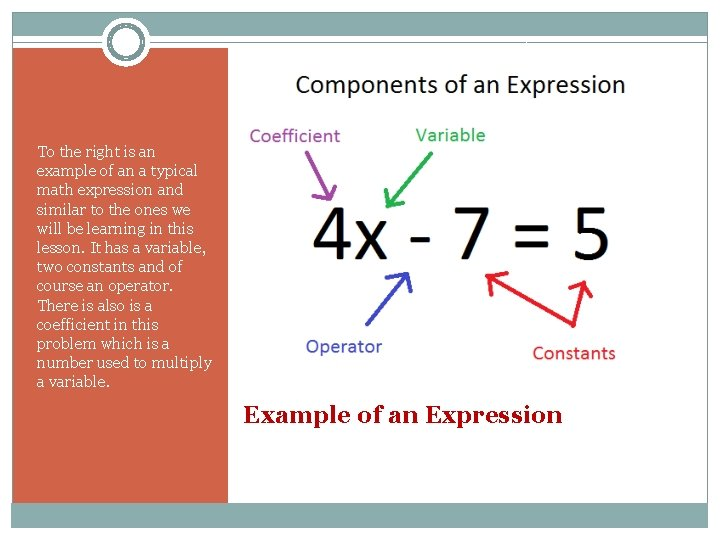To the right is an example of an a typical math expression and similar to the ones we will be learning in this lesson. It has a variable, two constants and of course an operator. There is also is a coefficient in this problem which is a number used to multiply a variable. Example of an Expression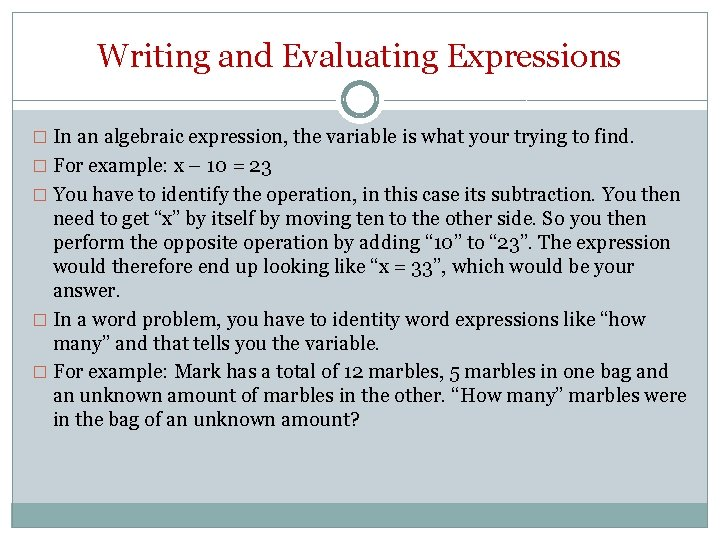Writing and Evaluating Expressions � In an algebraic expression, the variable is what your trying to find. � For example: x – 10 = 23 � You have to identify the operation, in this case its subtraction. You then need to get “x” by itself by moving ten to the other side. So you then perform the opposite operation by adding “ 10” to “ 23”. The expression would therefore end up looking like “x = 33”, which would be your answer. � In a word problem, you have to identity word expressions like “how many” and that tells you the variable. � For example: Mark has a total of 12 marbles, 5 marbles in one bag and an unknown amount of marbles in the other. “How many” marbles were in the bag of an unknown amount?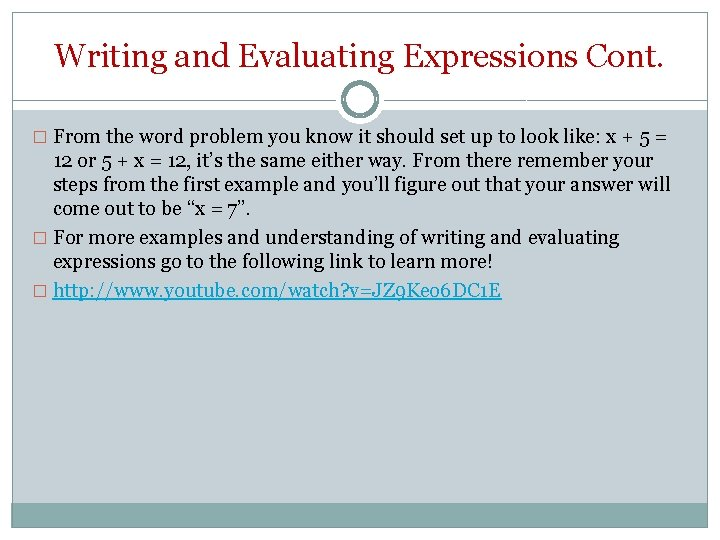Writing and Evaluating Expressions Cont. � From the word problem you know it should set up to look like: x + 5 = 12 or 5 + x = 12, it’s the same either way. From there remember your steps from the first example and you’ll figure out that your answer will come out to be “x = 7”. � For more examples and understanding of writing and evaluating expressions go to the following link to learn more! � http: //www. youtube. com/watch? v=JZ 9 Keo 6 DC 1 E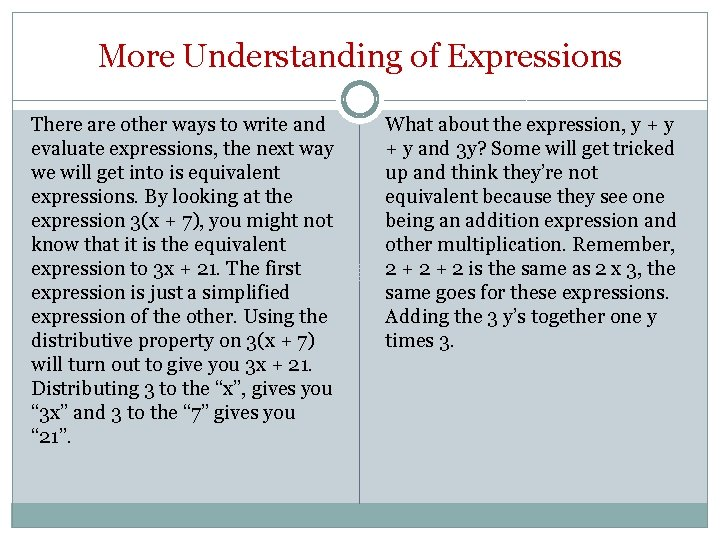More Understanding of Expressions There are other ways to write and evaluate expressions, the next way we will get into is equivalent expressions. By looking at the expression 3(x + 7), you might not know that it is the equivalent expression to 3 x + 21. The first expression is just a simplified expression of the other. Using the distributive property on 3(x + 7) will turn out to give you 3 x + 21. Distributing 3 to the “x”, gives you “ 3 x” and 3 to the “ 7” gives you “ 21”. What about the expression, y + y and 3 y? Some will get tricked up and think they’re not equivalent because they see one being an addition expression and other multiplication. Remember, 2 + 2 is the same as 2 x 3, the same goes for these expressions. Adding the 3 y’s together one y times 3.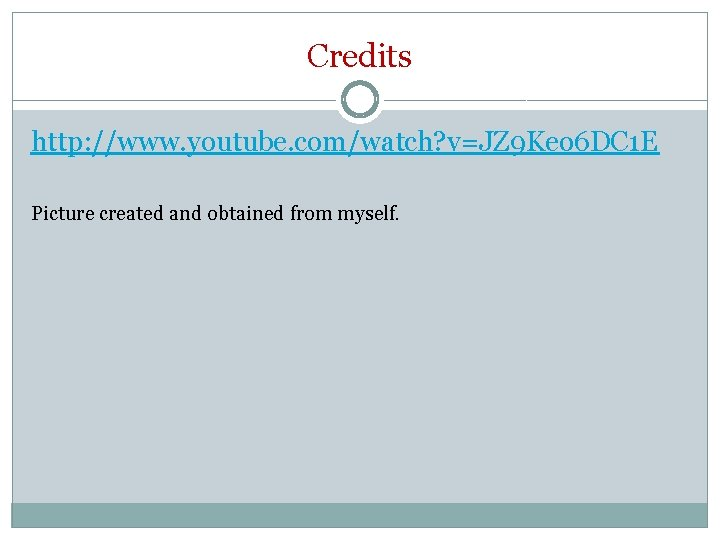Credits http: //www. youtube. com/watch? v=JZ 9 Keo 6 DC 1 E Picture created and obtained from myself.